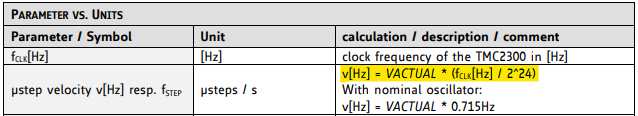Views:
The internal clock oscillator on the TMC2300 has a listed typical frequency of 12MHz and a frequency range of 9MHz to 15MHz at room temperature (see DC and Timing Characteristics tables in the datasheet for the TMC2300).

When using VACTUAL to command motor rotation (using the integrated STEP Pulse generator), the actual velocity of the motor depends on the speed of the internal clock.As such, if exact control over velocity is required, it is recommended to measure the clock frequency experimentally rather than assuming that the clock is operating at the typical 12MHz. The procedure to measure the internal clock frequency can be performed as follows:
• Turn on the TMC2300
• Turn off the motor by turning off the EN pin or setting enabledrv (CHOPCONF) to 0.
• Set diag_index in GCONF to 1
• Set a velocity value in the VACTUAL register
• Measure the time delta between two index pulses on the DIAG pin
• Calculate the fClk frequency from the measurement:

Microstep frequency generated by VACTUAL :
µSteps/s = VACTUAL * fClk / 2^24

One Index pulse gets generated every 4 fullsteps :
4 Fullsteps per Index pulse
mres µSteps per Fullstep
-> 1 index pulse per 4*mres µSteps

The index pulse frequency (f_Index) is therefore:
f_Index = µSteps/s / (4*mres) = VACTUAL * fClk / 2^24 / (4*mres)
= VACTUAL * fClk / 2^26 / mres

Setting a VACTUAL value of V_CALIBRATION and measuring the time period between two index pulses t_Index gives the clock frequency:
fClk = 2^26 * mres / V_CALIBRATION / t_Index

Example measurement:
When setting VACTUAL to 1000 and mres to 256, a measurement of 1.3215s between index pulses would indicate a clock frequency of ~13MHz.

Once the clock frequency is determined, you can re-calculate the required value for VACTUAL that should be used to rotate your motor at your desired speed.
Do keep in mind that the clock frequency can vary over temperature. If this needs to be accounted for, you can perform this measurement at multiple different temperatures to get a more precise idea of how the clock frequency changes over temperature.